# Multiplying out of gamut colors in the unbounded sRGB color space produces meaningless results

Occasionally a software developer will conclude that because unbounded sRGB (also called "extended sRGB") can be used to encode and display all possible colors, therefore it's also suitable for use as a universal working space for image editing. However, multiplying out of gamut colors in unbounded RGB color spaces produces physically impossible and mathematically meaningless results. As multiplication is a critically important part of image editing, unbounded sRGB can't be used as a universal working space for image editing.

Written May 2014.

## Introduction: Unbounded sRGB is OK for displaying but not for multiplying out of gamut colors

Unbounded sRGB (sometimes called "extended" sRGB) allows the encoding of otherwise out of gamut colors by using at least one channel and possible two RGB values that are less than zero and at least one channel that is greater than zero and might be greater than 1.0.

Dividing color A by color B is the same as multiplying color A by the inverse of color B. So this article only discusses multiplication. When multiplying colors in the unbounded sRGB color space, there are three possible cases:

1. Both colors are out of gamut with respect to the bounded sRGB working space xy chromaticities.
2. One color is out of gamut and the other is in gamut with respect to the bounded sRGB working space xy chromaticities.
3. Both colors are in gamut with respect to the bounded sRGB working space xy chromaticities.

When multiplying an out-of-gamut color with an in-gamut color, and also when multiplying two out-of-gamut colors, the resulting color might be:

• A color that's too bright and has the wrong hue.
• A color that's not only out of gamut with respect to the bounded sRGB chromaticities, but also out of gamut with respect to the image's source color space (multiplying in-gamut colors in any RGB working space never produces out of gamut colors).
• A physically impossible color that's technically in gamut with respect to the bounded sRGB chromaticities, but has a negative Luminance.
• A physically impossible and completely imaginary color that has a negative Luminance.

Occasionally a software developer will conclude that because unbounded sRGB (also called "extended sRGB") can be used to encode and display all possible colors, therefore it's also suitable as a "universal working space" for editing all images. Unfortunately, in the unbounded sRGB color space multiplying and dividing colors that are out of gamut with respect to the bounded sRGB color space produces meaningless results. Multiplying and dividing one color by another color is used in quite a few critically important image editing operations, including modifying an image's white balance. Therefore the unbounded sRGB color space is not suitable for image editing.

High bit depth GIMP 2.9 from git is perhaps unusual among image editors in that it actually does allow editing images that have been converted to the unbounded sRGB color space.

The default GIMP from git uses linear gamma RGB values for some editing operations and uses "gamma corrected" RGB values (using the default sRGB almost gamma=2.2 tone reproduction curve) for other editing operations. For images presented on this page I compiled and used a version of GIMP from git that I modified to ensure that all editing operations were done using linear gamma processing.

I also removed clipping code from selected editing operations, including, obviously, the Multiply blend mode. In case it's not so obvious why I removed the clipping code, if I had left the clipping code in place then the result of multiplying two colors would have been bounded by 0.0 and 1.0 and so would always be in gamut with respect to the bounded sRGB color space, which would completely invalidate the result of multiplying out of gamut colors. Either way, clipped or unclipped, multiplying out of gamut colors in the unbounded sRGB color space produces meaningless results.

## Multiplication in display-referred and scene-referred image editing produces normal, expected results

### Unbounded sRGB image editing compared to normal display-referred and scene-referred image editing

Unlike unbounded sRGB image editing, in display- and scene-referred image editing the user chooses the appropriate RGB working space for the task at hand, and that RGB working space is bounded, meaning out of gamut colors are neither used in nor generated by image processing:

1. For display-referred image editing, "bounded" means the RGB channel values are never allowed to be less than 0.0 or greater than 1.0. Consequently all possible combinations of RGB channel values produce colors that are in gamut with respect to the user-chosen RGB working space, and no editing operation produces colors that are out of gamut.
2. For scene-referred image editing, "bounded" means the RGB channel values are allowed to be as high as is needed to capture and manipulate high dynamic range scene data. But the RGB channel values are never less than 0.0. So again, all possible combinations of RGB channel values produce colors that are in gamut with respect to the user-chosen RGB working space, and no editing operation produces colors that are out of gamut. 1

### A boringly obvious summary of multiplication in display-referred and scene-referred image editing

The results of multiplying two colors in display-referred and scene-referred image editing are so boringly obvious, expected, and predictable as to normally not require any kind of discussion or summarization. But the results of multiplying colors that are outside the bounded sRGB color gamut while editing in unbounded sRGB are so bizarrely nonobvious and unexpected as to require a baseline for comparison. So here it is:

1. Multiplying two colors in normal scene- and display-referred image editors never produces a color that is outside the user-chosen RGB working space's color gamut (display-referred) or triangle of xy values created by drawing lines connecting the working space's chromaticities (scene-referred).
2. When multiplying two colors in a display-referred RGB working space, all RGB channel values are bounded by the range 0.0 to 1.0. Consequently, when multiplying Color1 and Color2, the resulting Color3 is either equal to or darker than both Color1 and Color2. This is just basic arithmetic:
• 0.0 times 0.0 is 0.0
• 1.0 times 1.0 is 1.0
• Multiplying any two floating point values between 0.0 and 1.0 produces a value that is smaller than either value, because a fractional part of a fractional part is smaller than the original fractional parts.
3. When multiplying two colors in a scene-referred RGB working space, all RGB channel values are greater than or equal to 0.0. The resulting color depends on the channel values of both colors:

## Multiplication in the unbounded sRGB color space produces physically impossible and mathematically meaningless results

The result of multiplying two colors in unbounded sRGB depends on whether or not one or both colors are out of gamut with respect to the bounded sRGB working space chromaticities. As stated above, encoding an out of gamut color in the unbounded sRGB color space requires using at least one and possibly two negative RGB channel values. As multiply is commutative (a times b equals b times a), there are three cases:

1. Color1 and Color2 are both out of gamut with respect to the bounded sRGB working space xy chromaticities.
2. Color1 is out of gamut and Color2 is in gamut with respect to the bounded sRGB working space xy chromaticities.
3. Color1 and Color2 are both in gamut with respect to the bounded sRGB working space xy chromaticities.

Considering all possible subcases, Case 1 and Case 2 can be reduced to three possible outcomes each, and Case 3 reduces to two possible outcomes. Cases 1 and 2 produce meaningless results. Case 3 reduces to normal display- or scene-referred results.

For each outcome presented below, colors that are out of gamut with respect to the bounded sRGB working space are from the linear gamma Rec. 2020 working space. Each outcome presents results from multiplying colors in the Rec. 2020 bounded working space vs multiplying the same colors in the unbounded sRGB color space ("same colors" means colors that are encoded in different RGB color spaces but have the same location in the XYZ/xyY reference color spaces):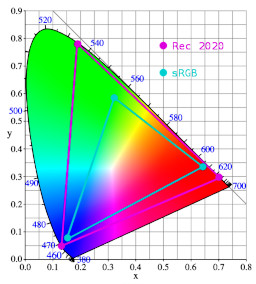1. The resulting colors and RGB channel values in the Rec. 2020 bounded working space vs the sRGB unbounded color space.
2. The locations of the resulting colors on a chromaticity diagram showing the triangles that indicate the locations of all possible bounded Rec. 2020 colors vs bounded sRGB colors.

With Case 3, where both colors are in gamut with respect to the sRGB chromaticities, it makes sense to distinguish between colors that have at least one channel value that is greater than 1.00 and colors for which all channel values are less than or equal to 1.00. This is not true for Cases 1 and 2. Unbounded sRGB image editing is neither scene-referred nor display-referred; consequently a channel value that is greater than 1.0 might mean "brighter", but also might mean "more saturated".

### Both colors are out of gamut with respect to the bounded sRGB xy chromaticities (Case 1)

In Case 1, Color1 and Color2 are both out of gamut with respect to the sRGB color space or chromaticities, so at least one channel for each color is negative. There are several subcases and combinations thereof to consider:

• At least one channel for both Color1 and Color2 must be negative. There are three subcases:
1. Two channels for Color1 might be negative and only one channel for Color2.
2. Two channels for Color2 might be negative and only one channel for Color1.
3. Color1 and Color2 are both negative in only one channel.
• At least one channel each for Color1 and Color2 must be positive. There are four subcases:
1. The positive channel(s) for Color1 might (both) be <=1.0.
2. The positive channel(s) for Color2 might (both) be <=1.0.
3. One or both of the positive channel(s) for Color1 might be >1.0.
4. One or both of the positive channel(s) for Color2 might be >1.0.
• At most one channel value for Color1 and Color2 can equal zero.
• Resulting channel values can be positive, negative, or zero:
1. If a positive channel value in Color1 corresponds to a positive channel value in Color2, the corresponding channel value for Color3 is positive (a positive times a positive is positive).
2. The negative channel(s) might correspond, meaning Color1 and Color2 are negative in the same channel(s). In this case the corresponding channel value(s) for Color3 is (are) positive (a negative times a negative is positive).
3. If a positive channel value in Color1 corresponds to a negative channel value in Color2, or vice versa, the corresponding channel value(s) for Color3 are negative (a positive times a negative is negative).
4. If a negative or positive channel value in Color1 corresponds to a zero channel value in Color2 or vice versa, the corresponding channel value(s) for Color3 is/are zero (any number times zero is zero).

Considering all possible channel values that result from multiplying Color1 and Color2, both of which are of gamut with respect to the sRGB chromaticities, there are three possible outcomes (at least; possibly I overlooked other outcomes). The resulting Color3 might be:

1. Negative in one or two or three channels, and zero in any remaining channel(s). The resulting Color3's xy value is in gamut with respect to the sRGB xy chromaticities, and the Y value is negative, representing perhaps a black hole that only exerts a gravitational pull on light of a particular color.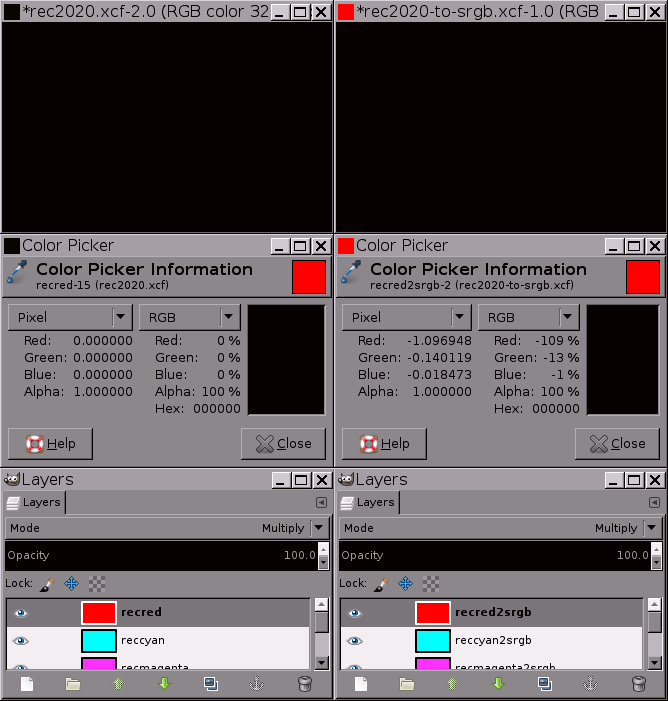In the unbounded sRGB color space, multiplying the out of gamut colors Rec. 2020 Red and Rec. 2020 Cyan produces a physically impossible saturated red-orange "color" with a negative luminance of Y = -0.345629. In the Rec. 2020 color space multiplying these two colors produces solid black.
2. Negative in one or two channels and positive in the remaining channel(s), and hence out of gamut with respect to the bounded sRGB xy values on the chromaticity diagram. There are four possible outcomes (two possibilities times two more possibilities):
1. The resulting out of gamut color might have a positive luminance (Y value) or might have a negative luminance (Y value).
2. The resulting out of gamut color might be a real color or might be an imaginary color.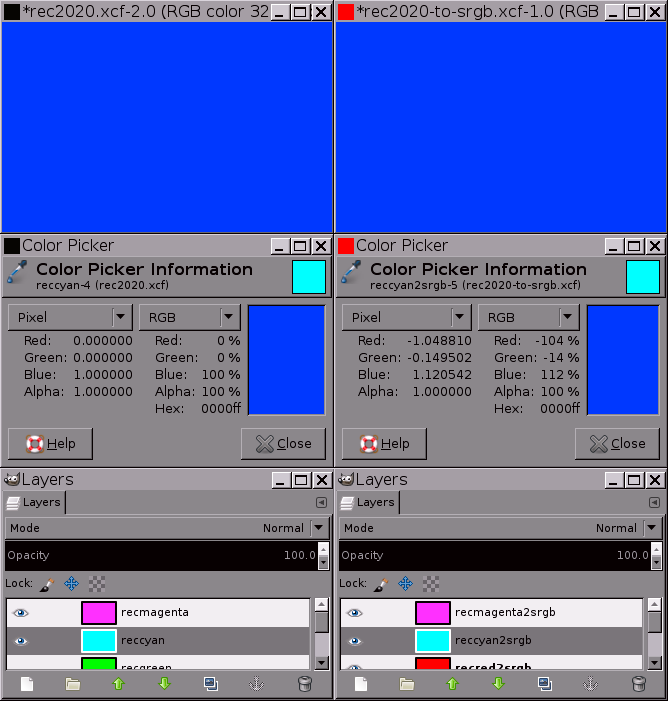In the unbounded sRGB color space, multiplying the out of gamut colors Rec. 2020 Magenta and Rec. 2020 Cyan produces a physically impossible and completely imaginary saturated blue "color" with a negative luminance of Y = -0.2726. In the Rec. 2020 color space multiplying these two colors produces Rec. 2020 Blue, exactly as expected.
3. Greater than or equal to zero in all three channels. In this case Color3 is in gamut with respect to the bounded sRGB xy values on the chromaticity diagram.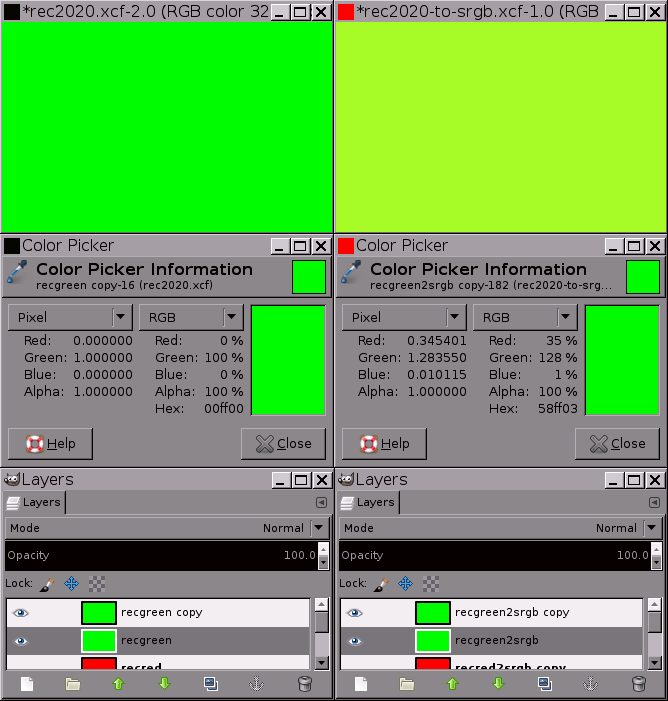In the unbounded sRGB color space, multiplying the out of gamut colors Rec. 2020 Green by Rec. 2020 Green produces a color that is brighter than Rec. 2020 Green and also a different shade of Green. In the Rec. 2020 color space multiplying Rec. 2020 Green by Rec. 2020 Green produces Rec. 2020 Green, exactly as expected.

### One color is in gamut with respect to the bounded sRGB xy chromaticities, and the other color is not (Case 2)

In Case 2, Color1 is out of gamut and Color2 is in gamut with respect to the bounded sRGB working space xy chromaticities. Considering the possible cases:

• Color1, which is outside the bounded sRGB color gamut:
1. Necessarily has at least one negative RGB channel value.
2. Might have two negative channel values, or might have one zero channel value and one negative channel value.
3. Must have at least one positive RGB channel value and might have two positive RGB channel values.
• Color2, which is inside the bounded sRGB color gamut, necessarily has three RGB channel values that are greater than or equal to zero.
• Resulting channel values can be positive, negative, or zero:
1. Multiplying any number by zero produces zero. So in Color3, channel value(s) equal to zero will result from multiplying Color1's channel value(s) by Color2's corresponding zero channel value(s), if any, and from multiplying Color2's channel values by Color1's corresponding zero channel value, if any.
2. Multiplying two positive numbers produces a positive number. So in Color3, the channel value(s) that result from multiplying Color1's positive channel value(s) by Color2's corresponding positive channel value(s) will be positive.
3. Multiplying a negative number by a positive number produces a negative number. So in Color3, any channel value(s) that result(s) from multiplying Color1's negative channel value(s) by Color2's corresponding positive channel value(s) will be negative.

Considering all possible channel values that result from multiplying the out-of-gamut Color1 and the in-gamut Color2 to produce Color3, there are three possible outcomes (at least; possibly I overlooked other outcomes):

1. If the in-gamut Color2 is zero in the channel(s) of the out-of-gamut Color1 that are greater than zero and positive in one or both of the channel(s) of the out-of-gamut Color1 that are less than zero, then the resulting color has one or two channel values that are equal to 0.0; with the remaining channel values being less than 0.0. This is a physically impossible color with a negative Y value, perhaps metaphorically equivalent to a black hole exerting a gravitational pull on passing lightwaves.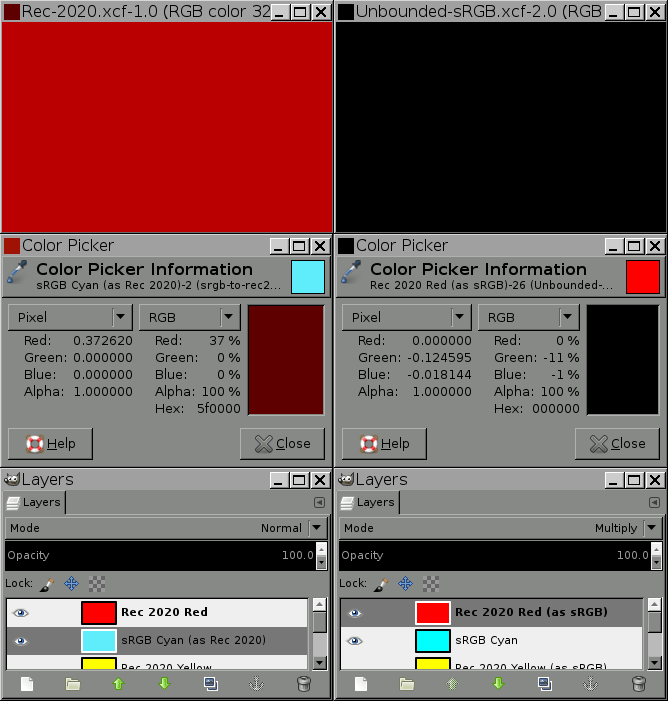Multiplying Rec. 2020's brightest, most saturated possible red and sRGB's brightest, most saturated possible cyan in the Rec 2020 bounded working space versus the sRGB unbounded working space: Above Left: In the Rec. 2020 color space above on the left, both colors are in gamut and the result of multiplying the two colors is also in gamut. As expected, in the Rec. 2020 color space, multiplying Rec. 2020 red (1.00, 0.00, 0.00) by sRGB cyan, which in the Rec. 2020 color space is (0.37, 0.93, 0.98), produces a darker shade of Rec. 2020 red, namely (0.37, 0.00, 0.00). Above Right: In the unbounded sRGB color space above on the right, Rec. 2020 red has the unbounded and out-of-gamut channel values (1.66, -0.12, -0.02). sRGB cyan has the in-gamut channel values (0.00, 1.00, 1.00). The result of multiplying the two colors in the unbounded sRGB color space is (0.00, -0.12, -0.02). This physically impossible "color" has a negative luminosity of Y = (0.00*0.22) + (-0.12*0.72) + (-0.02*0.06) = -0.09. Multiplying Rec. 2020 Red and sRGB Cyan in the bounded Rec. 2020 color space produces a dark shade of red, exactly as expected. Multiplying the same two colors in the unbounded sRGB color space produces a physically meaningless saturated cyan-green color with a negative luminance of Y=-0.09.
2. If the in-gamut Color2 is equal to zero in (both of) the channel(s) that correspond to Color1's negative channel value(s), and greater than or equal to zero in the channel(s) that correspond to Color1's positive channel, the resulting Color3 will be in gamut with respect to the bounded sRGB color space and will have a Y value that is greater than or equal to 0.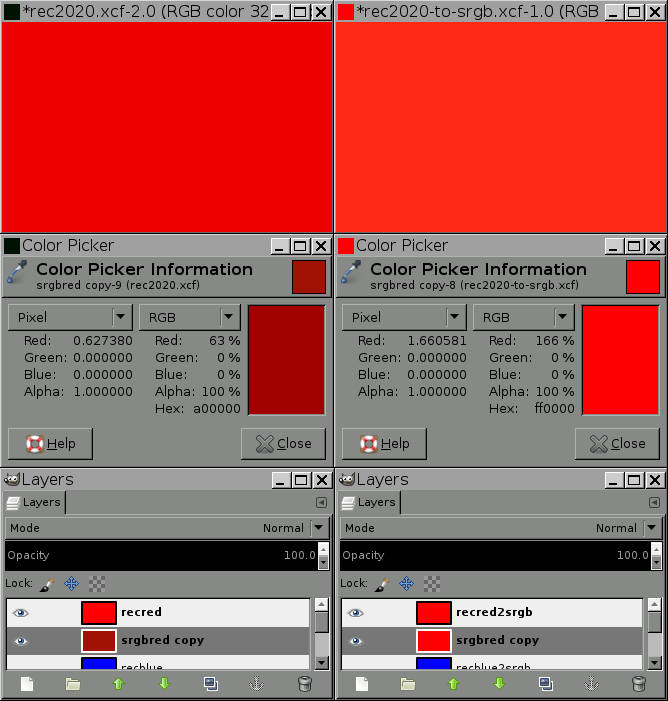Multiplying Rec. 2020's brightest, most saturated possible red and sRGB's brightest, most saturated possible red in the Rec 2020 bounded working space versus the sRGB unbounded working space: Above Left: In the Rec. 2020 color space above on the left, both colors are in gamut and the result of multiplying the two colors is also in gamut. As expected, in the Rec. 2020 color space, multiplying Rec. 2020 red (1.00, 0.00, 0.00) by sRGB red, which in the Rec. 2020 color space is (0.627380, 0.069117, 0.0.06389), produces a darker shade of Rec. 2020 red, namely (0.627380, 0.00, 0.00). The color's location on the chromaticity diagram is shown by the white dot in the lower right corner with the smaller red dot in the middle, which is of course the chromaticity for Rec. 2020 Red. The Luminance value is Y = 0.175063. Above Right: In the unbounded sRGB color space above on the right: Rec. 2020 red has the unbounded and out-of-gamut channel values (1.660581, -0.124595, -0.018144). sRGB red has the in-gamut channel values (1.00, 0.00, 0.00). The result of multiplying the two colors in the unbounded sRGB color space is (1.660581, 0.00, 0.00), which is in gamut with respect to the sRGB bounded color gamut and brighter than both sRGB red and Rec 2020 red, having a luminance of Y = 0.369460. This particular result might not seem like such an awful result. But if the artist were trying to work on either a display-referred or a scene-referred Rec. 2020 image, s/he would not be happy to find that multiplying Rec. 2020 Red (1.00, 0.00, 0.00) by sRGB Red (which is (0.627380, 0.069117, 0.0.06389) in the Rec. 2020 working space) produces a red that is brighter than Rec. 2020 Red (for which Y = 0.279037), also brighter than sRGB Red (for which Y = 0.222488), and is also less saturated and more orange than Rec. 2020 Red.
3. If the in-gamut Color2 is greater than zero in at least one channel that corresponds to (either of) Color1's negative channel value(s), but not in both of the channels that corresponds to Color1's positive channel(s), Color3 will be out of gamut with respect to the bounded sRGB color space. It might or might not have a positive Y value. For this particular case, I'll show an example where the resulting color has a positive Y value, and a second example where the resulting color has a negative Y value and is in fact an imaginary color, this time however, without also showing the corresponding chromaticity diagrams.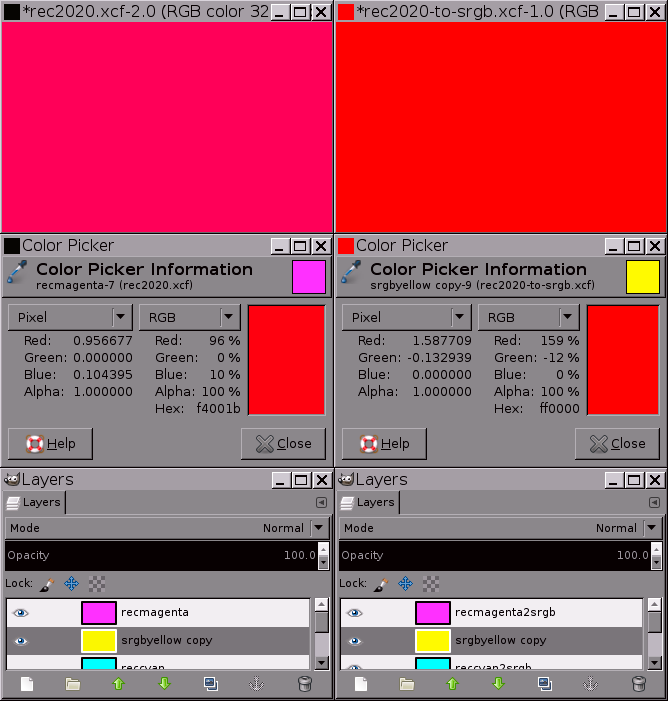Above on the left: Multipling Rec. 2020 Magenta by sRGB Yellow in the Rec. 2020 working space produces a bright saturated slightly magenta red for which Y = 0.271710. Above on the right: Multiplying the same two colors in the unbounded sRGB color space produces a very bright red for which Y = 0.257942. This red is out of gamut with respect to the bounded sRGB color space and also out of gamut with respect to the Rec. 2020 working space. This, of course, is an entirely unacceptable result to an artist who is trying to work with a display-referred Rec. 2020 image. Above on the left: Multiplying Rec. 2020 Cyan by sRGB Magenta in the Rec. 2020 working space produces a bright, saturated slightly cyan blue, for which Y = 0.095945. Above on the right: Multiplying the same two colors in the unbounded sRGB color space produces an imaginary saturated blue for which Y = -0.272612. This physically impossible and completely imaginary "color" is of course outside the bounded color gamuts of both the sRGB and Rec. 2020 working spaces. Multiplying Rec. 2020 Cyan by sRGB Magenta in the unbounded sRGB color space produces absurdly meaningless results.

### Both colors are in gamut with respect to the bounded sRGB working space xy chromaticities (Case 3)

Color1 and Color2 are both in gamut with respect to the bounded sRGB xy chromaticities. So all of the Color1 and Color2 channel values are >=0.0. There are two subcases:

1. If all the channel values in Color1 and Color2 are also <=1.0, then Color3, the product of multiplying Color1 and Color2 in the unbounded sRGB working space, will have the same channel values as would result from multiplying Color1 and Color2 in the display-referred (hence bounded) sRGB working space.
2. If any of the Color1 and Color2 channel values are >1.0, then Color3, the product of multiplying Color1 and Color2 in the unbounded sRGB working space, will have the same channel values as would result from multiplying Color1 and Color2 in the scene-referred (hence bounded) sRGB working space.

## Conclusion: Unbounded sRGB is a flawed model for image editing

Unbounded sRGB allows the encoding of colors that are otherwise out of gamut with respect to sRGB by using at least one channel value that is less than zero and at least one channel that is greater than zero and might be greater than 1.0.

Multiplication is an essential part of image editing. Among other uses, multiplication is use to change an image's white balance. However, the only colors for which multiplication in the unbounded sRGB color space gives rational, useable results in fact bounded sRGB colors, plus the limiting case of multiplying by gray. Multiplying out of gamut colors in the unbounded sRGB color space produces:

• Colors that are too bright and have the wrong hue.
• Colors that are not only out of gamut with respect to the bounded sRGB chromaticities, but also out of gamut with respect to the image's source color space.
• Physically impossible "colors" that are technically in gamut with respect to the bounded sRGB chromaticities, but have a negative Luminance.
• Physically impossible and completely imaginary colors that have negative Luminances.

Multiplying out of gamut colors in the unbounded sRGB color space consistently produces meaningless results. Therefore unbounded sRGB is a flawed model for image editing.

Using unbounded sRGB for displaying and storing images might have useful applications. However, because multiplying out of gamut colors produces meaningless results, unbounded sRGB should never be used for image editing.

Notes:

• The exception would be as the direct result of a user-initiated editing action such as subracting a color (all RGB channel values being greater than or equal to 0.0) from solid black.